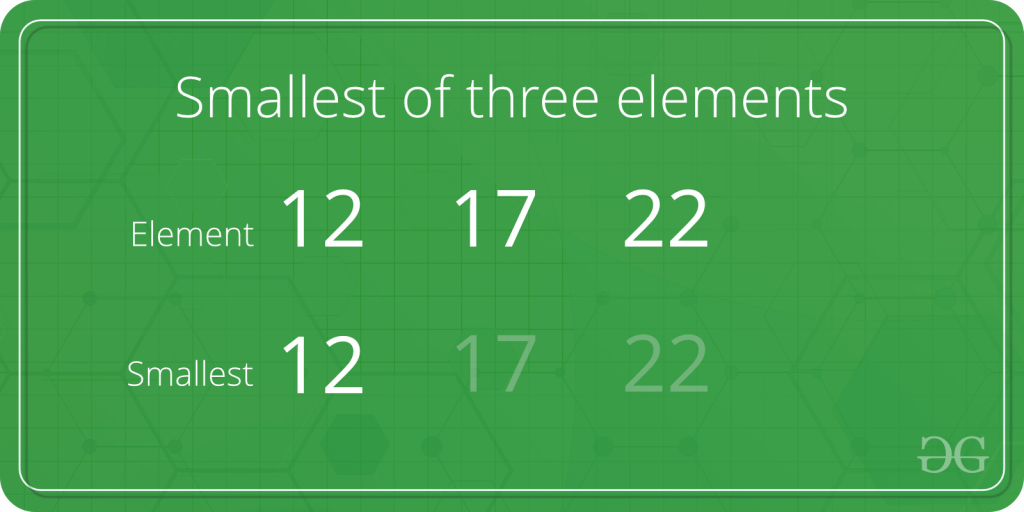Skip to content
Related Articles
Program to find the smallest element among three elements
• Last Updated : 11 May, 2021

Given three numbers. The task is to find the smallest among given three numbers.Examples:

```Input: first = 15, second = 16, third = 10
Output: 10

Input: first = 5, second = 3, third = 6
Output: 3```

Approach:

1. Check if the first element is smaller than second and third. If yes then print it.
2. Else if check if the second element is smaller than first and third. If yes then print it.
3. Else third is the smallest element and print it.

Below is the implementation of the above approach:

## C++

 `// C++ implementation to find``// the smallest of three elements``#include ``using` `namespace` `std;` `int` `main()``{``    ``int` `a = 5, b = 7, c = 10;` `    ``if` `(a <= b && a <= c)``        ``cout << a << ``" is the smallest"``;` `    ``else` `if` `(b <= a && b <= c)``        ``cout << b << ``" is the smallest"``;` `    ``else``        ``cout << c << ``" is the smallest"``;` `    ``return` `0;``}`

## Java

 `// Java implementation to find``// the smallest of three elements` `import` `java.io.*;` `class` `GFG {``  `   `    ``public` `static` `void` `main (String[] args) {``            ``int` `a = ``5``, b = ``7``, c = ``10``;` `    ``if` `(a <= b && a <= c)``        ``System.out.println( a + ``" is the smallest"``);` `    ``else` `if` `(b <= a && b <= c)``        ``System.out.println( b + ``" is the smallest"``);` `    ``else``        ``System.out.println( c + ``" is the smallest"``);` `    ``}``}``// This code is contributed by shs..`

## Python3

 `# Python implementation to find``# the smallest of three elements` `a, b, c ``=` `5``, ``7``, ``10` `if``(a <``=` `b ``and` `a <``=` `c):``    ``print``(a, ``"is the smallest"``)` `elif``(b <``=` `a ``and` `b <``=` `c):``    ``print``(b, ``"is the smallest"``)``else``:``    ``print``(c, ``"is the smallest"``)` `# This code is contributed``# by 29AjayKumar`

## C#

 `// C# implementation to find``// the smallest of three elements``using` `System;` `class` `GFG``{``static` `public` `void` `Main ()``{``int` `a = 5, b = 7, c = 10;``if` `(a <= b && a <= c)``    ``Console.WriteLine( a + ``" is the smallest"``);` `else` `if` `(b <= a && b <= c)``    ``Console.WriteLine( b + ``" is the smallest"``);` `else``    ``Console.WriteLine( c + ``" is the smallest"``);``}``}` `// This code is contributed by jit_t`

## PHP

 `

## Javascript

 ``
Output:
`5 is the smallest`

Time complexity : O(1)

Attention reader! Don’t stop learning now. Get hold of all the important DSA concepts with the DSA Self Paced Course at a student-friendly price and become industry ready.  To complete your preparation from learning a language to DS Algo and many more,  please refer Complete Interview Preparation Course.

In case you wish to attend live classes with industry experts, please refer DSA Live Classes

My Personal Notes arrow_drop_up